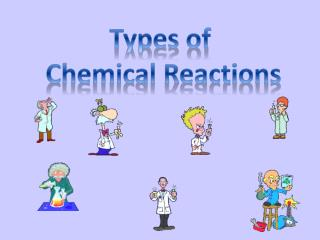DownloadDownload PresentationTypes of Chemical Reactions

# Types of Chemical Reactions

Télécharger la présentation## Types of Chemical Reactions

- - - - - - - - - - - - - - - - - - - - - - - - - - - E N D - - - - - - - - - - - - - - - - - - - - - - - - - - -
##### Presentation Transcript

1. Types of Chemical Reactions

2. Synthesis Reactions • In a synthesis reaction, also known as a composition reaction, two or more substances combine to form a new compound. • This type of reaction is represented by the following general equation: • A + X → AX • A and X can be elements or compounds. • AX is a compound.

3. To visualize a synthesis reaction, look at the cartoon above. • A skinny bird (reactant) and the worm (reactant) combine to make one product, the fat bird.

4. Two or more reactants yielding one product is another way to identify a synthesis reaction. 2Mg (s) + O2 (g) → 2MgO (s) two or more reactants one product

5. Example of a Synthesis Reaction

6. Decomposition Reactions • In a decomposition reaction, a single compound undergoes a reaction that produces two or more simpler substances. • Decomposition reactions are the opposite of synthesis reactions. • They are represented by the following general equation: • AX → A + X • AX is a compound. • A and X can be elements or compounds.

7. To visualize a decomposition reaction, look at the cartoon above. • In this cartoon, the egg (reactant) which contained the turtle at one time, now has opened and the turtle (product) and egg shell (product) are now two separate substances.

8. A reaction with only one reactant and more than one product is another way to identify a decomposition reaction. H2CO3 (aq) → CO2 (g) + H2O (l) one reactant more than one product

9. Example of a Decomposition Reaction

10. Single-Displacement Reactions • In a single-displacement reaction, also known as a replacement reaction, one element replaces a similar element in a compound. • Many single-displacement reactions take place in aqueous solution. • Single-displacement reactions can be represented by the following general equations. • A + BX → AX + B or Y + BX → BY + X • A, B, X, and Y are elements. AX, BX, and BY are compounds.

11. To visualize a single-displacement reaction, look at the above cartoon. • Notice the guy in the orange shirt steals the date of the other guy. • So, a part of one of the reactants trades places and is in a different place among the products.

12. Example: 2Al (s) + 3Pb(NO3)2 (aq) → 3Pb (s) + 2Al(NO3)3 (aq) When solid aluminum is placed in a solution of lead(II) nitrate, the aluminum replaces the lead. Solid lead and aqueous aluminum nitrate are formed. Notice that the reactants are an element and a compound and the products are an element and a compound.

13. Example of a Single-Displacement Reaction

14. Double-Displacement Reactions • In double-displacement reactions, the ions of two compounds exchange places in an aqueous solution to form two new compounds. • One of the compounds formed is usually a precipitate, an insoluble gas that bubbles out of the solution, or a molecular compound, usually water. • The other compound is often soluble and remains dissolved in solution.

15. A double-displacement reaction is represented by the following general equation. • AX + BY → AY + BX • A, X, B, and Y in the reactants represent ions. • AY and BX represent ionic or molecular compounds.

16. To visualize a double-displacement reaction, look at the cartoon above. • Notice the “hats” have exchanged places.

17. Example: formation of a precipitate 2KI (aq) + Pb(NO3)2 (aq) → PbI2 (s) + 2KNO3 (aq) “partners trade places” Lead(II) iodide

18. Example: formation of a gas FeS (s) + 2HCl (aq) → H2S (g) + FeCl2 (aq) “partners trade places”

19. Example: formation of water (molecular) HCl (aq) + NaOH (aq) → NaCl (aq) + H2O (l) “partners trade places”

20. Combustion Reactions • In a combustion reaction, a substance combines with oxygen, releasing a large amount of energy in the form of heat and light.

21. Examples: 2H2 (g) + O2 (g) → 2H2O (g) C3H8 (g) + 5O2 (g) → 3CO2 (g) + 4H2O (g) propane